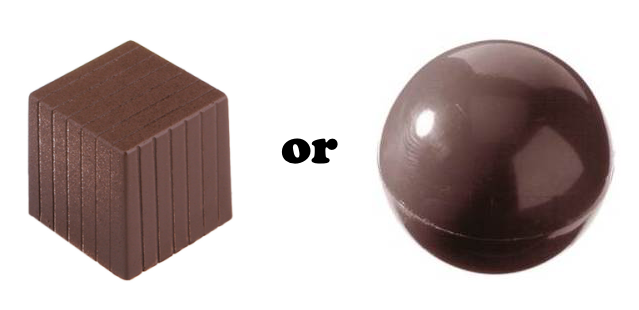# Solid Chocolate Puzzle

Geometry Level 1A solid sphere of chocolate has a volume of $288\pi$. The chocolate is then melted down and reshaped into a solid cube. Assume no chocolate was wasted.

Did the sphere have a greater surface area or does the cube?

Useful equations:

• Volume of a sphere: $V=\frac{4}{3}\pi r^3$
• Volume of a cube: $V=a^3$
• Surface area of a sphere: $A=4\pi r^2$
• Surface area of a cube: $A=6a^2$
×